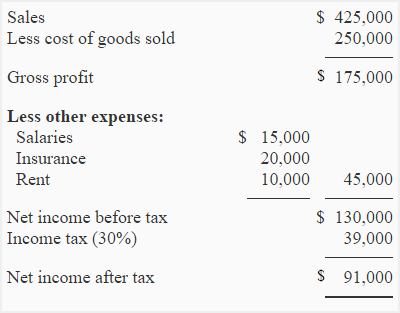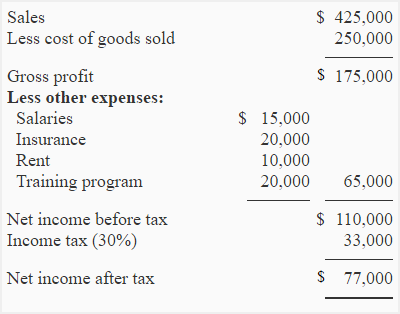# Exercise-19 (After-tax cost computation)

The projected income statement of Eastern company is given below:Eastern company wants to start a training program that would cost \$20,000. The training program is a tax deductible expense. The tax rate of  Eastern company is 30%.

Required:

1. Prepare a new projected income statement of Eastern company to show the effect of training program on net operating income and income tax.
2. What would be the after-tax cost of training program.

## Solution:

### (1) Projected income statement with training program:### (2) Computation of after-tax cost of training program:

Because the training program is a tax deductible expense, it would reduce the taxable income of the company by \$20,000 and reduce the income tax by \$6,000 (\$39,000 – 33,000). The after-tax cost of training program would, therefore, be \$14,000 (\$20,000 – \$6,000).

Alternatively, we can compute the after-tax cost easily by using after-tax cost formula:

After-tax cost = (1 – tax rate) × Tax deductible expense

= (1 – 0.3) × \$20,000

= 0.7 × \$20,000

= \$14,000

A D V E R T I S E M E N T
One Comment on Exercise-19 (After-tax cost computation)
1.wice

how to get rm 30000 for training program?